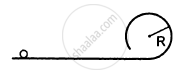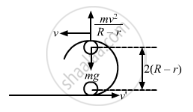Advertisement Remove all ads

# The Following Figure Shows a Rough Track, a Portion of Which is in the Form of a Cylinder of Radius R. - Physics

Sum

The following figure shows a rough track, a portion of which is in the form of a cylinder of radius R. With what minimum linear speed should a sphere of radius r be set rolling on the horizontal part so that it completely goes round the circle on the cylindrical part.Advertisement Remove all ads

#### SolutionLet the sphere be thrown with velocity $v'$ and its velocity becomes at the top-most point.

From the free body diagram of the sphere, at the topmost point, we have

$\frac{m v^2}{R - r} = mg$

$\Rightarrow v^2 = g\left( R - r \right)$

On applying the law of conservation of energy, we have

$\left( \frac{1}{2}m\nu '^2 + \frac{1}{2}I\omega '^2 \right) = 2mg\left( R - r \right) + \left( \frac{1}{2}m \nu^2 + \frac{1}{2}I \omega^2 \right)$

$\Rightarrow \frac{7}{10}m\nu '^2 = 2mg\left( R - r \right) + \frac{7}{10}m \nu^2$

$\Rightarrow \frac{7}{10}m\nu '^2 = 2mg\left( R - r \right) + \frac{7}{10}mg(R - r)$

$\Rightarrow \frac{7}{10}\nu '^2 = \frac{27g\left( R - r \right)}{10}$

$\Rightarrow \nu' = \sqrt{\frac{27}{7} g\left( R - r \right)}$

Concept: Momentum Conservation and Centre of Mass Motion
Is there an error in this question or solution?
Advertisement Remove all ads

#### APPEARS IN

HC Verma Class 11, 12 Concepts of Physics 1
Chapter 10 Rotational Mechanics
Q 78 | Page 200
Advertisement Remove all ads
Advertisement Remove all ads
Share
Notifications

View all notifications

Forgot password?